# Test: Electrical & Electronic Measurements- 4

## 10 Questions MCQ Test RRB JE for Electrical Engineering | Test: Electrical & Electronic Measurements- 4

Description
Attempt Test: Electrical & Electronic Measurements- 4 | 10 questions in 30 minutes | Mock test for Electrical Engineering (EE) preparation | Free important questions MCQ to study RRB JE for Electrical Engineering for Electrical Engineering (EE) Exam | Download free PDF with solutions
QUESTION: 1

### A 200 V, 5 A d.c. energy meter is tested at its marked ratings. The resistance of the pressure circuit is 8000 Ω and that of current coil is 0.1 Ω. The power consumed when testing the meter with phantom loading with current circuit excited by a 6 V battery is?

Solution:

Power consumed by current coil = VI = 6 × 5 = 30W
Power consumed by pressure coil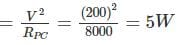Total power = 30 + 5 = 35 W

*Answer can only contain numeric values
QUESTION: 2

### The meter constant of a single phase 240V induction watt-hour meter is 500 revolutions per kWh. The sped of the meter disc for a current of 15A at 0.6 P.f lagging will be ------ (in rpm)

Solution:

P = VI cosϕ = 240 × 15 × 0.6 = 2160 W = 2.16 KW
Meter constant = 500 revolutions/kwh
⇒ For 2.16 kW = 1080 revolutions/hour
= 18 revolution/minute
∴ Speed of disc = 18 rpm

QUESTION: 3

### The braking torque provided by a permanent magnet in a single phase energy meter is proportional to the i) square of the flux of the permanent magnet ii) speed of the meter iii) distance of he permanent magnet from the center of the revolving disc Q. Which of the above statements are correct.

Solution:

The braking torque is provided by the interaction of the eddy current and the field of the permanent magnet. this torque is directly proportional to the product of square of flux of the magnet, magnitude of eddy current and the effective radius R from the axis of the disc. braking torque is directly proportional to speed of rotation.

QUESTION: 4

Which one of the following defects is responsible for creeping in an induction type energy meter?

Solution:

In some meters continuous rotation is obtained even when there is no current passing through the current coil and only pressure coil is energized. This is called creeping. The major cause for creeping is overcompensation for friction.

*Answer can only contain numeric values
QUESTION: 5

A 230 V, single phase watt hour meter has a constant load of 6 A passing through it for 8 hours at unity power factor. If the meter disc makes 2400 revolutions during this period. What is the power factor of the load if the number of revolutions made by the meter are 1800 when operating at 230 V and 8 A for 6 hours?

Solution:

Energy supplied = VI cos ϕ × t × 10-3
= 230 × 6 × 8 × 10-3 = 11.04 kwh
Meter constant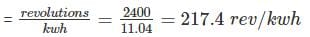Energy consumed when the meter takes 1800 revolutions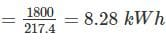Now energy consumed = VI cos ϕ × t × 10-3
8.28 = 230 × cos ϕ × 8 × 6 × 10-3
⇒ cos ϕ = 0.75

*Answer can only contain numeric values
QUESTION: 6

In a vibrating reed frequency meter the natural frequencies of two adjacent reeds have a difference of

Solution:

The vibrating reed frequency meter, originally employed by Békésy and later by Wilson as a cochlear model, uses a set of tuned reeds to represent the cochlea's graded bank of resonant elements and an elastic band threaded between them to provide nearest-neighbour coupling.

QUESTION: 7

The constant for a three phase, 3 element integrating wattmeter is 0.12 revolution of disc per kWh. If the meter is normally used with a potential transformer of ratio 22,000/110V and a current transformer of ratio 500/5A; find the error expressed as a percentage of the correct reading from the following test figures for the instrument only
Line voltage = 100 V; current = 5.25 A; power factor = 1
Time to complete 40 revolutions = 61s.

Solution:

Actual energy consumed during the test period = √3 × ratio of P.T. × ratio of C.T. × VsIs cosϕ × t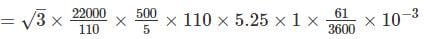= 338.97 kWh
Energy recorded by meter during the test period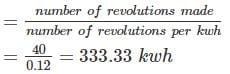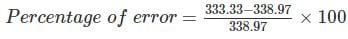= −1.66% = 1.66% low

QUESTION: 8

A single phase energy meter operating on 220 V and 8 A for half day i.e. 12 hours makes 3200 revolutions. If the meter constant = 600 revolutions/kwh, then the power factor of the load will be

Solution:

Actual energy consumed = VI cos ϕ × t × 10-3 kwh where t is in hours
Energy recoded = 3200/600 = 5.33kwh
⇒ 220 × 8 × 12 × cos ϕ × 10-3 = 5.33
Cos ϕ = 0.2525

*Answer can only contain numeric values
QUESTION: 9

A 220 V, single phase, watt hour meter has a constant load of 4 A passing through it for 6 hour at unity power factor. The meter disc makes 2376 revaluation during this period. If the number of revolution made by the meter are 1542 when operating at 220 V and 6 A for 4 hours, then power factor of the load would be

Solution: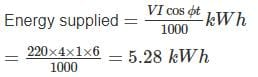Energy meter constant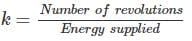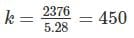Energy consumed when the meter make 1542 revaluations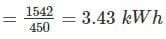Now energy consumed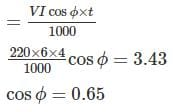QUESTION: 10

A 220V, 10 A dc energy meter is tested for its name plate ratings. Resistance of the pressure coil circuit is 8000 Ω and that of current coil itself is 0.12 Ω calculate the energy consumed when testing for a period of 1 hour with phantom loading with the current coil circuit excited by a separated 9 V battery.

Solution: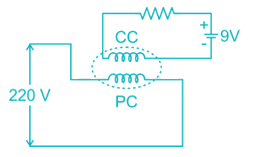Power consumed in the pressure coil circuit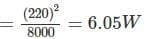Power consumed in the current coil circuit = 9 × 10 = 90 W
Total energy consumed with direct measure = 96.05 W
Total energy consumed during 1 hour with direct measurement = 96.05 × 1 = 96.05 WhUse Code STAYHOME200 and get INR 200 additional OFF Use Coupon Code